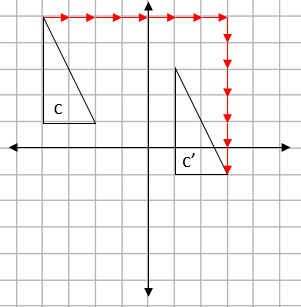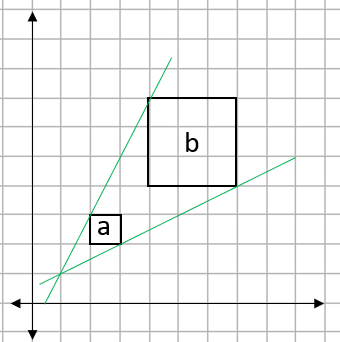For full tracking and unlimited access to thousands of activities for £1 today.

• ## Accessibility

Open the accessibility toolbar to change fonts and contrast, access a dictionary, use a ruler and more

There are three transformations that will allow an object to keep the same sides and angles (this is properly called congruency).

Rotation, Reflections and Translations.

Sometimes, you will be given a transformed object and be asked to describe the transformation.

What do you need to give to describe a transformation?

Each transformation has a different list of information that you must give.

• Reflection - You need to give the equation of the mirror line.
• Translation - You need to give the vector
• Rotation - You need to give the distance, direction and centre of rotation.

What do I need to say first?

The thing that is often missed out when students complete these questions is which transformation it is! The first thing you should always state is if the transformation is a reflection, rotation or a translation.

Congruent Vs Similar

If a shape doesn't change its angles or sides it is congruent
If a shape doesn't change its angles but does change its sides, it is similar.

If we apply this to transformations;

Translations, reflections and rotations will produce congruent shapes.

Enlargements will produce similar shapes.

Example 1: Describe this transformation fully.Step 1: Decide which transformation this is.

a to a' is flipped. this means that the transformation is a reflection.

Step 2: Give the information we need.

For a reflection, we need a mirror line. This will always be exactly half way between the two shapes.This mirror line goes through the x axis at -2. This means the mirror line is x = -2

Example 2: Describe this transformation fully.Firstly, we can see that this is a rotation.The easiest way to find the centre of rotation is to trace the original shape and just try some points, when you find the one that works, that is the centre.

This transformation is described by...

Distance: 90°

Direction: Clockwise

Centre: (0,0)

Example 3: Describe this transformation fully.We can see that this is a translation.To describe a translation. We have to give a vector.

We can see from the arrows that are drawn that this is a movement of 7 to the right and 6 down.

This gives the vector...

 7 -6

Example 4: Shape A is enlarged to shape B. Find the scale factor and centre of enlargement.Finding the Scale Factor.

All we have to do here is to look at one of the pairs of sides. If we use the top of each shape, shape A is 1cm long and shape B is 3 cm long.

This gives a scale factor of 3.

Finding the centre of enlargement.

To find this, we join up two pairs of matching corners with striaght lines and see where they join up.We can see here that the two lines join up at the point (1,1). This is the centre of enlargement.

10 questions